﻿ 带积分型源项二阶拋物型方程的参数识别问题 An Inverse Problem of Identifying the Coefficient of Second Order Parabolic Equation with Integral Source Term

Vol. 09  No. 03 ( 2020 ), Article ID: 34684 , 9 pages
10.12677/AAM.2020.93047

An Inverse Problem of Identifying the Coefficient of Second Order Parabolic Equation with Integral Source Term

Yujuan Zheng

School of Mathematics and Physics, Lanzhou Jiaotong University, Lanzhou Gansu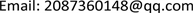Received: Mar. 1st, 2020; accepted: Mar. 16th, 2020; published: Mar. 24th, 2020ABSTRACT

This paper mainly studies an inverse problem of identifying the coefficient of second order parabolic equation with integral source term. We use the optimal control framework to establish control functional and prove the existence, and the necessary conditions of the minimum for the control functional are established. Since the optimal control problem is nonconvex, one may not expect a unique solution. However in this paper the solution is proved to be locally unique and stable.

Keywords:Inverse Problem, Optimal Control, Parameter Identification, Well-Posedness1. 引言(1.1)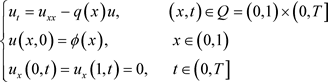(1.2)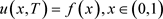(1.3)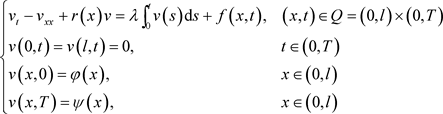(1.4)

2. 最优控制问题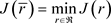(2.1)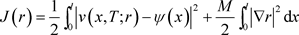(2.2)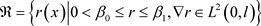(2.3)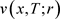是问题(1.4)的解，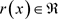，M是正则化系数，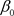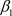是两个已知正常数。(2.4)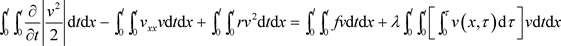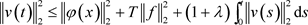3. 存在性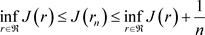,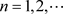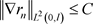(3.1)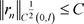(3.2)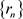的子序列仍记为，使得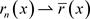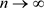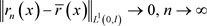(3.3)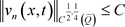(3.4)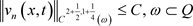(3.5)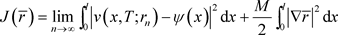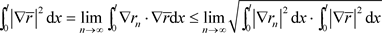4. 必要条件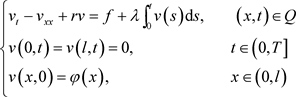(4.1)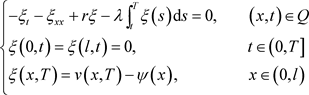(4.2)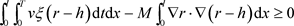(4.3)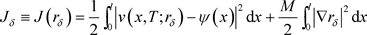(4.4)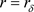时令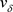为(1.4)的解，由于r是最优解则有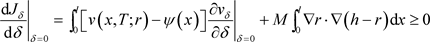(4.5)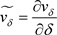计算得出如下方程：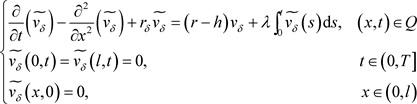(4.6)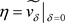满足(4.7)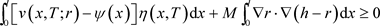(4.8)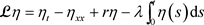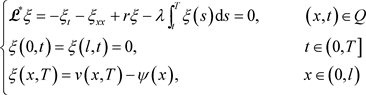(4.9)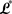的伴随算子，由(4.7)和(4.9)式可得：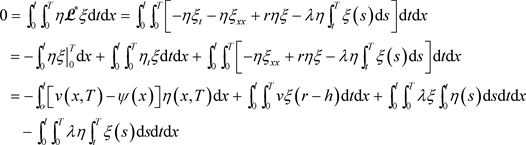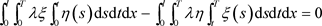(4.10)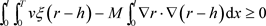5. 局部唯一性和稳定性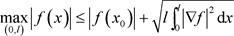(5.1)(5.2)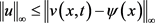(5.3)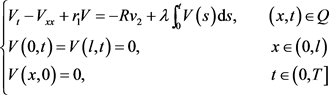(5.4)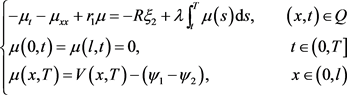(5.5)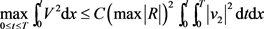(5.6)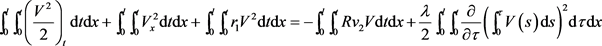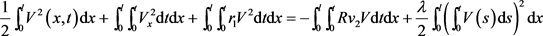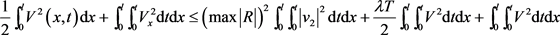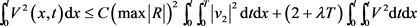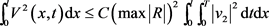C是T与无关的常数。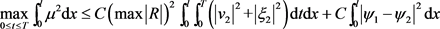(5.7)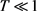时由Gronwall’s不等式可得：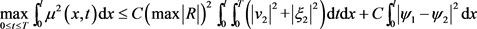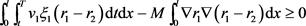(5.8)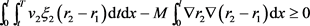(5.9)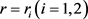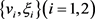分别是(4.1)，(4.2)的解(5.10)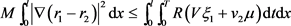(5.11)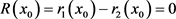(5.12)(5.13)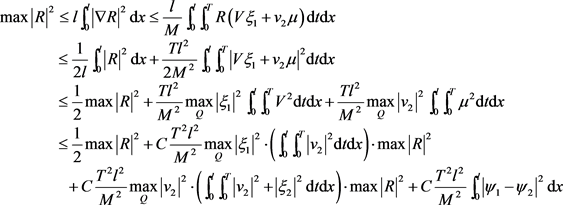(5.14)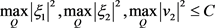(5.15)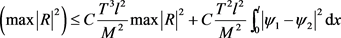(5.16)使(5.17)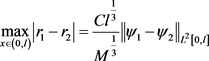(5.18)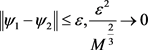An Inverse Problem of Identifying the Coefficient of Second Order Parabolic Equation with Integral Source Term[J]. 应用数学进展, 2020, 09(03): 391-399. https://doi.org/10.12677/AAM.2020.93047

1. 1. 周蜀林. 偏微分方程[M]. 北京: 北京大学出版社, 2005.

2. 2. 姜礼尚, 等, 编. 数学物理方程讲义[M]. 北京: 高等教育出版社, 1996.

3. 3. Aboulaichb, R. and Achchaba, D. (2018) Parameter Identification by Optimization Method for a Pollution Problem in Porous Media. Acta Mathematica Scientia, 38, 1345-1360. https://doi.org/10.1016/S0252-9602(18)30818-X

4. 4. Yang, L., Yu, J.-N. and Deng, Z.-C. (2008) An Inverse Problem of Identifying the Coefficient of Parabolic Equation. Applied Mathematical Modelling, 32, 1984-1995. https://doi.org/10.1016/j.apm.2007.06.025

5. 5. Li, Z.L. and Zheng. K.W. (1998) An Inverse Problem in a Parabolic Equation. Differential Equations and Computational Simulation, 6, 203-209.

6. 6. 杨柳. 具奇异或退化性质的二阶拋物型方程的系数反演问题[D]: [博士学位论文]. 兰州: 兰州大学, 2016.

7. 7. Prilepko, A.I., Orlovsky, D.G. and Vasin, I.A. (2000) Methods for Solving Inverse Problems in Mathematical Physics. Vol. 1. Marcel Dekker, New York.

8. 8. Deng, Z.C. and Yang, L. (2014) An Inverse Problem of Identifying the Radiative Coefficient in a Degen-erate Parabolic Equation. Chinese Annals of Mathematics, 35B, 355-382. https://doi.org/10.1007/s11401-014-0836-x